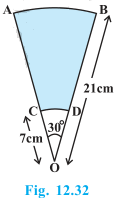# AB and CD are respectively arcs of two concentric circles of radii 21 cm and 7 cm and centre O (see Fig.) . If ∠AOB = 30°, find the area of the shaded region.Solution

Radius of the larger circle, R = 21 cm

Radius of the smaller circle, r = 7 cm

Angle made by sectors of both concentric circles = 30°

Area of shaded region=Area of sector AOB-Area of sector COD

=θ/(360°) πR2-θ/(360°) πr2

=θ/(360°) π[R2-r2]

=30°/360*22/7[(21)2-(7)2]

=1/12*22/7*28*14

=308/3cm2

=102.66cm2

The area of shaded region 102.66cm2